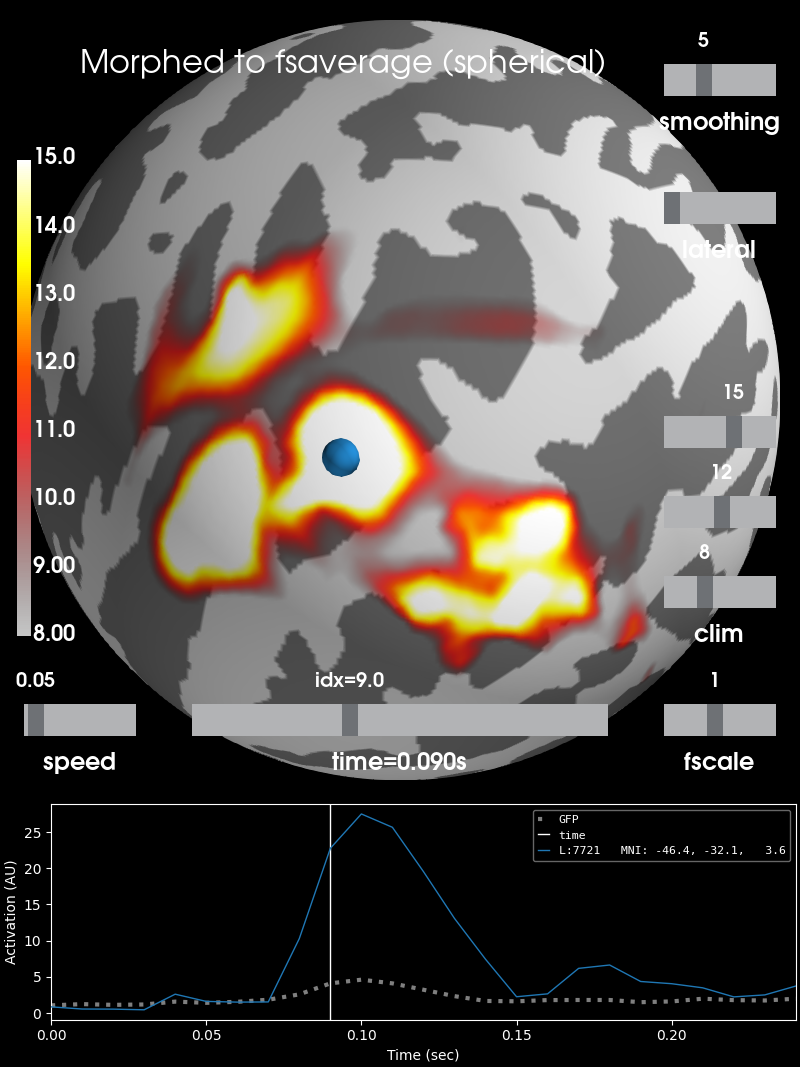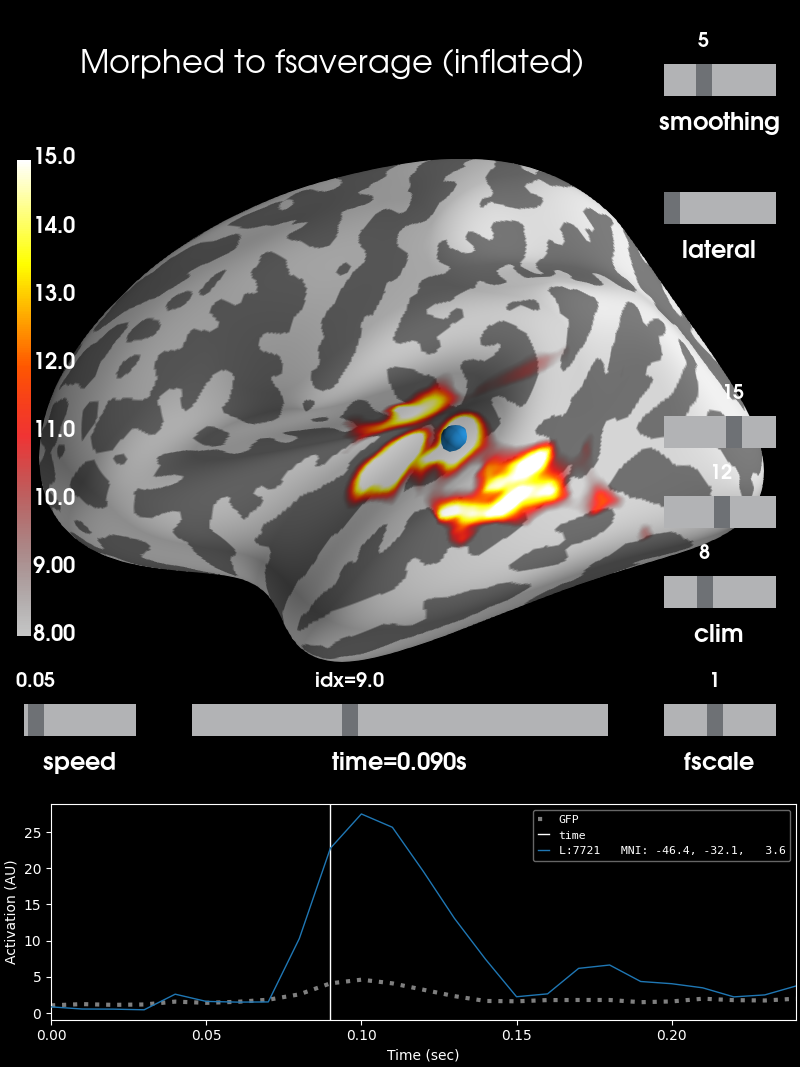# Morph surface source estimate¶

This example demonstrates how to morph an individual subject’s `mne.SourceEstimate` to a common reference space. We achieve this using `mne.SourceMorph`. Pre-computed data will be morphed based on a spherical representation of the cortex computed using the spherical registration of FreeSurfer (https://surfer.nmr.mgh.harvard.edu/fswiki/SurfaceRegAndTemplates) 1. This transform will be used to morph the surface vertices of the subject towards the reference vertices. Here we will use ‘fsaverage’ as a reference space (see https://surfer.nmr.mgh.harvard.edu/fswiki/FsAverage).

The transformation will be applied to the surface source estimate. A plot depicting the successful morph will be created for the spherical and inflated surface representation of `'fsaverage'`, overlaid with the morphed surface source estimate.

Note

For background information about morphing see Morphing and averaging source estimates.

```# Author: Tommy Clausner <tommy.clausner@gmail.com>
#
import os
import os.path as op

import mne
from mne.datasets import sample

print(__doc__)
```

Setup paths

```data_path = sample.data_path()
sample_dir = op.join(data_path, 'MEG', 'sample')
subjects_dir = op.join(data_path, 'subjects')
fname_src = op.join(subjects_dir, 'sample', 'bem', 'sample-oct-6-src.fif')
fname_fwd = op.join(sample_dir, 'sample_audvis-meg-oct-6-fwd.fif')
fname_fsaverage_src = os.path.join(subjects_dir, 'fsaverage', 'bem',
'fsaverage-ico-5-src.fif')

fname_stc = os.path.join(sample_dir, 'sample_audvis-meg')
```

```# Read stc from file
```

## Setting up SourceMorph for SourceEstimate¶

In MNE, surface source estimates represent the source space simply as lists of vertices (see The SourceEstimate data structure). This list can either be obtained from `mne.SourceSpaces` (src) or from the `stc` itself. If you use the source space, be sure to use the source space from the forward or inverse operator, because vertices can be excluded during forward computation due to proximity to the BEM inner skull surface:

```src_orig = mne.read_source_spaces(fname_src)
print(src_orig)  # n_used=4098, 4098
print(fwd['src'])  # n_used=3732, 3766
print([len(v) for v in stc.vertices])
```

Out:

```    Reading a source space...
Computing patch statistics...
[done]
Computing patch statistics...
[done]
<SourceSpaces: [<surface (lh), n_vertices=155407, n_used=4098>, <surface (rh), n_vertices=156866, n_used=4098>] MRI (surface RAS) coords, subject 'sample', ~27.4 MB>
Computing patch statistics...
[done]
Computing patch statistics...
[done]
Desired named matrix (kind = 3523) not available
Read MEG forward solution (7498 sources, 306 channels, free orientations)
Source spaces transformed to the forward solution coordinate frame
<SourceSpaces: [<surface (lh), n_vertices=155407, n_used=3732>, <surface (rh), n_vertices=156866, n_used=3766>] head coords, subject 'sample', ~31.0 MB>
[3732, 3766]
```

We also need to specify the set of vertices to morph to. This can be done using the `spacing` parameter, but for consistency it’s better to pass the `src_to` parameter.

Note

Since the default values of `mne.compute_source_morph()` are `spacing=5, subject_to='fsaverage'`, in this example we could actually omit the `src_to` and `subject_to` arguments below. The ico-5 `fsaverage` source space contains the special values `[np.arange(10242)] * 2`, but in general this will not be true for other spacings or other subjects. Thus it is recommended to always pass the destination `src` for consistency.

Initialize SourceMorph for SourceEstimate

```src_to = mne.read_source_spaces(fname_fsaverage_src)
print(src_to['vertno'])  # special, np.arange(10242)
morph = mne.compute_source_morph(stc, subject_from='sample',
subject_to='fsaverage', src_to=src_to,
subjects_dir=subjects_dir)
```

Out:

```    Reading a source space...
[done]
[done]
[    0     1     2 ... 10239 10240 10241]
```

## Apply morph to (Vector) SourceEstimate¶

The morph will be applied to the source estimate data, by giving it as the first argument to the morph we computed above.

```stc_fsaverage = morph.apply(stc)
```

## Plot results¶

```# Define plotting parameters
surfer_kwargs = dict(
hemi='lh', subjects_dir=subjects_dir,
clim=dict(kind='value', lims=[8, 12, 15]), views='lateral',
initial_time=0.09, time_unit='s', size=(800, 800),
smoothing_steps=5)

# As spherical surface
brain = stc_fsaverage.plot(surface='sphere', **surfer_kwargs)

brain.add_text(0.1, 0.9, 'Morphed to fsaverage (spherical)', 'title',
font_size=16)
```As inflated surface

```brain_inf = stc_fsaverage.plot(surface='inflated', **surfer_kwargs)

brain_inf.add_text(0.1, 0.9, 'Morphed to fsaverage (inflated)', 'title',
font_size=16)
```## Reading and writing SourceMorph from and to disk¶

An instance of SourceMorph can be saved, by calling `morph.save`.

This method allows for specification of a filename under which the `morph` will be save in “.h5” format. If no file extension is provided, “-morph.h5” will be appended to the respective defined filename:

```>>> morph.save('my-file-name')
```

Reading a saved source morph can be achieved by using `mne.read_source_morph()`:

```>>> morph = mne.read_source_morph('my-file-name-morph.h5')
```

Once the environment is set up correctly, no information such as `subject_from` or `subjects_dir` must be provided, since it can be inferred from the data and use morph to ‘fsaverage’ by default. SourceMorph can further be used without creating an instance and assigning it to a variable. Instead `mne.compute_source_morph()` and `mne.SourceMorph.apply()` can be easily chained into a handy one-liner. Taking this together the shortest possible way to morph data directly would be:

```stc_fsaverage = mne.compute_source_morph(stc,
subjects_dir=subjects_dir).apply(stc)
```

For more examples, check out examples using SourceMorph.apply.